Home > PC > Chapter 11 > Lesson 11.1.3 > Problem11-49

11-49.
1. The binomial terms you found in parts (c) and (d) of the previous problem are called the complex conjugates of the complex number. You can use the complex conjugate to simplify division problems involving complex numbers. Simplify the following division problems by multiplying the numerator and the denominator by the complex conjugate of the denominator. Homework Help ✎

1.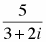2.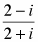3.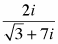4.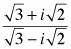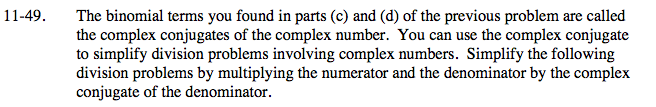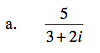$\frac{5}{3+2\textit{i}}\left( \frac{3-2\textit{i}}{3-2\textit{i}} \right)$

$\frac{15-10\textit{i}}{9-6\textit{i}+6\textit{i}-4\textit{i}^2}$

$\frac{15-10\textit{i}}{9+4}$

$\frac{15}{13}-\frac{10}{13}\textit{i}$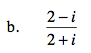$\frac{3}{5}-\frac{4}{5}\textit{i}$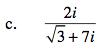$\frac{2\textit{i}}{\sqrt{3}+7\textit{i}}\left( \frac{\sqrt{3}-7\textit{i}}{\sqrt{3}-7\textit{i}} \right)$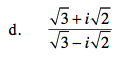$\frac{\sqrt{3}+\textit{i}\sqrt{2}}{\sqrt{3}-\textit{i}\sqrt{2}}\left( \frac{\sqrt{3}+\textit{i}\sqrt{2}}{\sqrt{3}+\textit{i}\sqrt{2}} \right)$